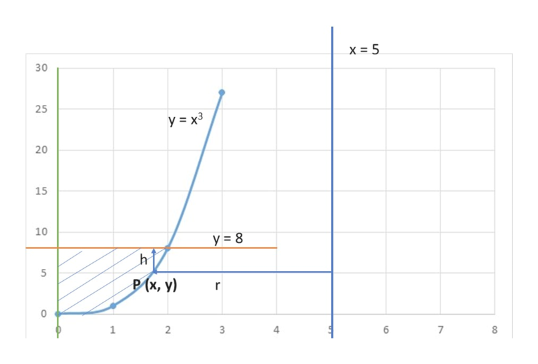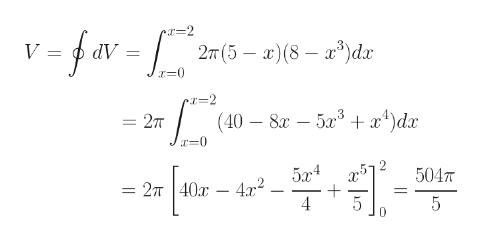# Use the method of cylindrical shells to find the volume generated by rotating the region bounded by the given curves about the specified axis.y = x3, y = 8, x = 0, about x = 5

Question
55 views

Use the method of cylindrical shells to find the volume generated by rotating the region bounded by the given curves about the specified axis.

y = x3, y = 8, x = 0, about x = 5

check_circle

Step 1

Please see the attached diagram on the white board. Please see it crefully and locate the lines, curve and points of interest. Please see the point P (x, y)

x = 5 is the axis of rotation. The cylindrical shell will have a volume, dV = 2πrh.dx

h = 8 - y = 8 - x3

r = 5 - x

Limits of integration will be x = 0 to x = 2help_outlineImage Transcriptionclosex 5 30 25 y=x3 20 15 10 y = 8 h P (x, y) r 0 8 2 3 4 6 7 fullscreen
Step 2

hence, dV = 2π(5 - x)(8 - x3)dx

Pleas...help_outlineImage Transcriptioncloser-2 2T (5 x(8)dx AP (40 8x 53+ x4)dx 27T 5x4 2т | 40х — 4? 4 5047 = 5 fullscreen

### Want to see the full answer?

See Solution

#### Want to see this answer and more?

Solutions are written by subject experts who are available 24/7. Questions are typically answered within 1 hour.*

See Solution
*Response times may vary by subject and question.
Tagged in

### Calculus